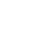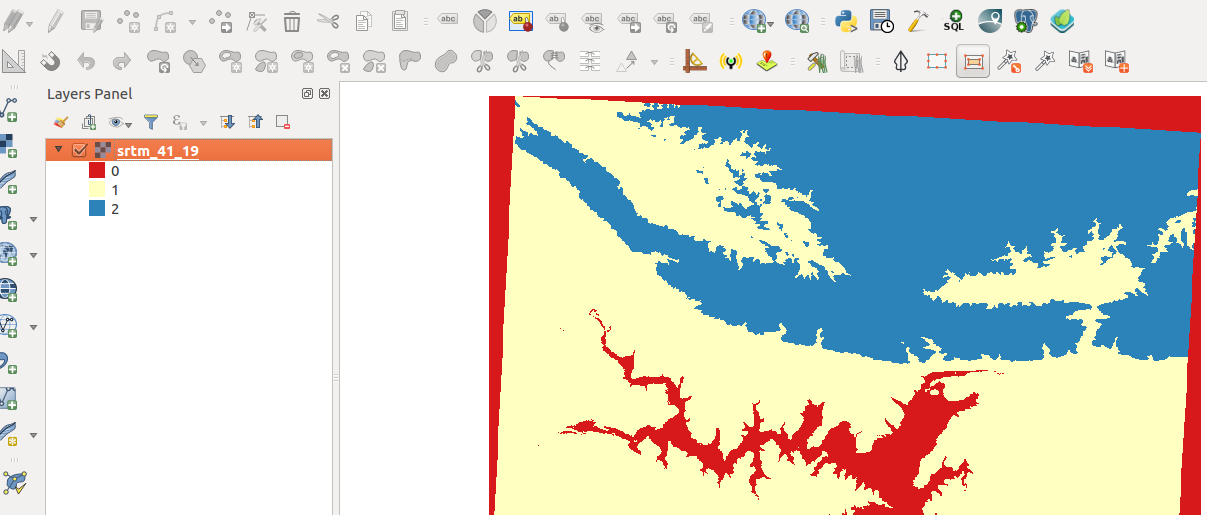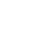### Raster calculator in Context

“Raster consists of pixels which are organised in rows and columns. Each pixel has got a values which represents a specific phenomenon on the earth surface. ”

Because of raster data is made up of cells it allows us to do arithmetic computations on the cells and generate new data. The most common raster operation is raster recode which allows us to generate a simplified raster layer ie converting a raster to a binary one.
In this module, we will look at how rasters can be manipulated by using arithmetic operations. Let's dig in and explore the raster calculator.#### You try:

Goal: To learn how to reclassify raster pixel values into 2 or 3 categories with raster calculator.

TODO

Produce a reclassified raster with 2 classes.
Advanced users Explore the possibility of creating 3 or more classes using the raster calculator.

• Use the raster calculator to create a binary raster where all pixels < 200 are reclassified to 1 and pixels> 200 are reclassified to 0. Use the binary recode expression specified below.
• Symbolize the raster you have generated above using the Unique Values render.

Reclassify the original raster to 3 classes with the following pixel ranges. Modify the Recode* expression to suit your needs.

Pixel Range New Value
Pixel_value < 200 0
Pixel_value > 200 and < 1000 1
Pixel_value >1000 2
• Symbolize the raster you have generated above using the Unique Values render.

Name Expectation

Binary recode

`("[email protected]" < 200) * 1`

Recode

`(("[email protected]" >= 5) AND ("srtm_41_19" <= 8))*3 + (("srtm_41_1" >= 9) AND ("srtm_41_1" <= 11))*2+(("srtm_41_1" >= 12) AND ("[email protected]" <= 15))*1`Raster analysis enforces its spatial relationships based on the location of the cell. Raster operations performed on multiple input raster datasets generally output cell
values that are the result of computations on a cell-by-cell basis. The value of the output for one cell is usually independent of the value or location of other input or output cells. In some cases, output cell values are influenced by neighbouring cells or groups of cells, such as in focal functions.

Raster calculator provides a means to do cell by cell analysis by using arithmetic operations and mathematical formulae to compute new outputs. Raster calculator functions mainly fall into the local operations category. These constitute the bulk of the analysis techniques that occur for rasters. The cell values of the new raster are computed by a function relating the input to output or are assigned by a classification table.1. Is the Unique Values render type specific to raster data only:
1. Yes
2. No
2. Which of these datasets can we apply arithmetic operations on:
1. A GIS raster dataset where each pixel represents the depth of a flood
2. A GIS vector dataset where each polygon represents an area of high, medium or low flood depth
3. A GIS vector dataset where each polygon represents a building with high, medium or low occupancy rate
3. Raster calculator can be used with Digital elevation models:
1. True
2. False